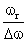Courses

# Test: Resonance In LCR Circuit

## 10 Questions MCQ Test Physics For JEE | Test: Resonance In LCR Circuit

Description
This mock test of Test: Resonance In LCR Circuit for JEE helps you for every JEE entrance exam. This contains 10 Multiple Choice Questions for JEE Test: Resonance In LCR Circuit (mcq) to study with solutions a complete question bank. The solved questions answers in this Test: Resonance In LCR Circuit quiz give you a good mix of easy questions and tough questions. JEE students definitely take this Test: Resonance In LCR Circuit exercise for a better result in the exam. You can find other Test: Resonance In LCR Circuit extra questions, long questions & short questions for JEE on EduRev as well by searching above.
QUESTION: 1

### A pure resistance R, pure capacitance C and pure inductance L are connected in series and the impedance of the circuit at resonance is Z0. If they are connected in parallel to each other, the maximum impedance at resonance will be:​

Solution:

If series LCR circuit is present then z={R2+(xl-xc)2}1/2
if resonance is present then XC=CL or VC=CL then Z= Zo
if LCR circuit is in parallel form then in which circuit resonance is also present firstly 1/Z ={(1/R)2+ ((1/xc) –(1/xl))2}1/2 and in resonance conditions XC=CL according to this Z= Zo then option C is the correct answer

QUESTION: 2

### At resonance the impedance of RLC circuit is

Solution:

Resonance is a condition in LCR circuit where the inductive reactance ( XL) and capacitive reactance (Xc) is equal.
Impedance at resonance is equal to the Resistance of the resistor because the other two cancel themselves off according to the below formula -
Z2=R2+(XL2-Xc2)
As XL = Xc ,
Z = R

QUESTION: 3

### Ratiois equal to

Solution:

frequency-to-bandwidth ratio of the resonator:
Q=fr/△f= ωr/△ω
where fr is the resonant frequency, Δf is the resonance width or full width at half maximum (FWHM) i.e. the bandwidth over which the power of vibration is greater than half the power at the resonant frequency, ωr = 2πfr is the angular resonant frequency, and Δω is the angular half-power bandwidth.

QUESTION: 4

When the oscillations produced are of constant amplitude. The are called

Solution:

If the losses incurred in the electrical system could be compensated, the amplitude of oscillation would remain constant and as such the oscillation would continue indefinitely against both external disturbances and changes in the initial conditions. This type of oscillation is called undamped oscillation.

QUESTION: 5

Find the series resonance frequency when L = 0.09 H, C = 25 F​

Solution:

ω=1/√LC
ω=1√0.09x25
ω=0.666
ω=2πf
f=0.666/(2 x 3.14)
f=0.106 s-1

QUESTION: 6

Measure of sharpness in an ac circuit is given by

Solution:
QUESTION: 7

In a series RCL circuit at resonance the applied ac voltage is 220 V. The potential drop across the inductance is 110V, what is the potential drop across the capacitor?​

Solution:

At resonance, the potential drop across the capacitance is equal and opposite to that of inductance i.e. 110V.

QUESTION: 8

Acceptor circuit is

Solution:

The series resonance circuit is known as an acceptor circuit. The impedance of the acceptor circuit is minimum but the voltage can be magnified.
The Acceptor circuit provides the maximum response to currents at its resonant frequency.
Series resonance circuit is known as acceptor circuit because the impedance at the resonance is at its minimum so as to accept the current easily such that the frequency of the accepted current is equal to the resonant frequency.

QUESTION: 9

An ac circuit contains a resistance R, capacitance C and inductance L in series with a source of emf ε = ε0sin (ωt – Ø). The current through the circuit is maximum when:​

Solution:
QUESTION: 10

Resonant circuits are used in tuning of

Solution:

The most common application of tank circuits is tuning radio transmitters and receivers. For example, when we tune a radio to a particular station, the LC circuits are set at resonance for that particular carrier frequency. A parallel resonant circuit can be used as load impedance in output circuits of RF amplifiers.